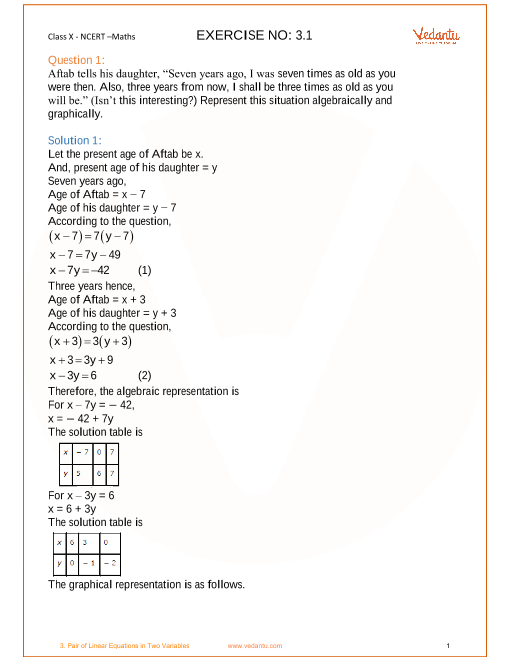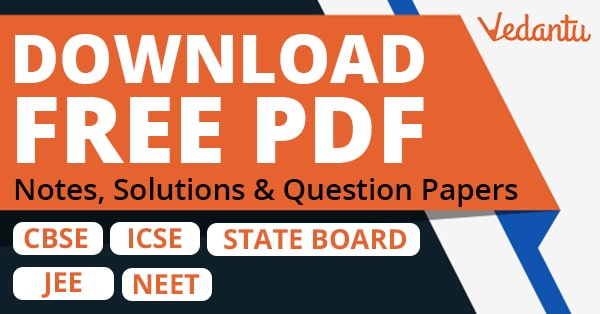# NCERT Solutions for Class 10 Maths Chapter 3 Pair of Linear Equations in Two Variables (Ex 3.4) Exercise 3.4

## NCERT Solutions for Class 10 Maths Chapter 3 Pair of Linear Equations in Two Variables (Ex 3.4) Exercise 3.4

Free PDF download of NCERT Solutions for Class 10 Maths Chapter 3 Exercise 3.4 (Ex 3.4) and all chapter exercises at one place prepared by expert teacher as per NCERT (CBSE) books guidelines. Class 10 Maths Chapter 3 Pair of Linear Equations in Two Variables Exercise 3.4 Questions with Solutions to help you to revise complete Syllabus and Score More marks. Register and get all exercise solutions in your emails.

You can also download NCERT Solutions for Class 10 Maths to help you to revise complete syllabus and score more marks in your examinantions